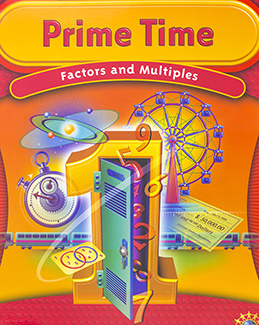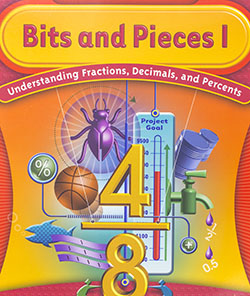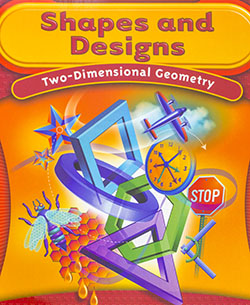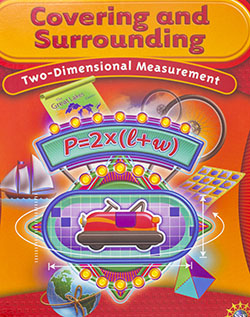Display Accessibility Tools

##Prime Time

In Prime Time students will explore certain important properties of whole numbers, especially those related to multiplication and division. The investigations in this unit will help students to:

• Understand relationships among factors, multiples, divisors, and products;
• Recognize and use properties of prime and composite numbers, even and odd numbers, and square numbers;
• Use rectangles to represent the factor pairs of numbers;
• Develop strategies for finding factors and multiples, least common multiples, and greatest common factors;
• Recognize and use the fact that every whole number can be written in exactly one way as a product of prime numbers;
• Use factors and multiples to solve problems and to explain some numerical facts of everyday life;
• Develop a variety of strategies for solving problems building models, making lists and tables, drawing diagrams, and solving simpler problems.

##Bits and Pieces I

In Bits and Pieces I students will investigate and make sense of rational numbers and operations with rational numbers. The investigations in this unit will help students to:

• Build an understanding of fractions, decimals, and percents and the relationships between and among these concepts and their representations;
• Develop ways to model situations involving fractions, decimals, and percents;
• Understand and use equivalent fractions to reason about situations;
• Compare and order fractions;
• Move flexibly between fraction, decimal, and percent representations;
• Use benchmarks such as 0, 1/2 , 1, and 1/4 to help estimate the size of a number or sum;
• Develop and use benchmarks that relate different forms of representations of rational numbers (for example, 50% is the same as 1/2 and 0.5);
• Use physical models and drawings to help reason about a situation;
• Look for patterns and describe how to continue the pattern;
• Use context to help reason about a situation;
• Use estimation to understand a situation.

##Shapes and Designs

Shapes and Designs was created to help students to:

• Understand some important properties of polygons and recognize polygonal shapes both in and out of the classroom;
• Investigate the symmetries of a shape-rotational or reflectional;
• Estimate the size of any angle using reference to a right angle and other benchmark angles;
• Use an angle ruler for making more accurate angle measurements;
• Explore parallel lines and angles created by lines intersecting parallel lines;
• Find patterns that help determine angle sums of polygons;
• Determine which polygons fit together to cover a flat surface and why;
• Explain the property of triangles that makes them useful as a stable structure for building;
• Reason about and solve problems involving shapes.

## Bits and Pieces II

Bits and Pieces II was created to help students make sense of one aspect of rational number: fraction operations. The investigations in this unit will help students to:

• Use benchmarks and other strategies to estimate the reasonableness of results of operations with fractions;
• Develop ways to model sums, differences, products, and quotients with areas, strips, and number lines;
• Use estimates and exact solutions to make decisions;
• Look for and generalize patterns in numbers;
• Use knowledge of fractions and equivalence of fractions to develop algorithms for adding, subtracting, multiplying and dividing fractions;
• Recognize when addition, subtraction, multiplication, and division is the appropriate operation to solve a problem;
• Write fact families to show the inverse relationship between addition and subtraction, and between multiplication and division;
• Solve problems using arithmetic operations on fractions;
• Look for and generalize patterns.

##Covering and Surrounding

In Covering and Surrounding student work with investigations leads them to:

• Understand area and relate area to covering a figure;
• Understand perimeter and relate perimeter to surrounding a figure;
• Develop strategies for finding areas and perimeters of rectangular shapes and non-rectangular shapes;
• Discover relationships between perimeter and area. including that each can vary while the other stays fixed;
• Understand how the areas of simple geometric figures relate to each other (e.g. the area of a parallelogram is twice the area of a triangle with the same base and height);
• Develop formulas and procedures-stated in words and/or symbols-for finding areas and perimeters of rectangles, parallelograms, triangles, and circles;
• Develop techniques for estimating the area and perimeter of an irregular figure;
• Recognize situations in which measuring perimeter or area will help answer practical questions.

## Bits and Pieces III

Bits and Pieces III, along with Bits and Pieces I and Bits and Pieces II, completes the development of meaning of, and operations on, fractions, decimals, and percents. This unit will help students to:

• Connect to what students already know about operations on fractions and whole numbers;
• Develop and use benchmarks and other strategies to estimate the answers to computations with decimals;
• Develop meaning of and algorithms for operations with decimals;
• Use the relationship between decimals and fractions to develop and understand why decimal algorithms work;
• Use the place value interpretation of decimals to make sense of short-cut algorithms for operations;
• Generalize number patterns to help make sense of decimal operations;
• Understand when addition, subtraction, multiplication or division is an appropriate operation to use to solve a problem;
• Understand that decimals are often associated with measurements in real world situations;
• Solve problems using operations on decimals;
• Use understanding of operations and the meaning of percents to solve percent problems of the form a% of b equals c for any one of the variables a, b, or c;
• Create and interpret circle graphs

##How Likely Is It?

The unit How Likely Is It? was created to help students:

• Understand that probabilities are useful for predicting what will happen over the long run
• Understand the concepts of equally likely and not equally likely;
• Understand that a game of chance is fair only if each player has the same chance of winning, not just a possible chance of winning;
• Understand that there are two ways to build probability models: by gathering data from experiments (experimental probability) and by analyzing the possible equally likely outcomes (theoretical probability);
• Understand that experimental probabilities are better estimates of theoretical probabilities when they are based on larger numbers of trials;
• Develop strategies for finding both experimental and theoretical probabilities;
• Critically interpret statements of probability to make decisions or answer questions.

The unit Data About Us was created to help students:

• Understand and use the process of data investigation by posing questions, collecting and analyzing data distributions, and making interpretations to answer questions;
• Represent data distributions using line plots, bar graphs, stem-and-leaf plots, and coordinate graphs;
• Compute the mean, median, or mode and the range of the data;
• Distinguish between categorical data and numerical data and identify which graphs and statistics may be used to represent each kind of data;
• Make informed decisions about which graph or graphs and which of the measures of center (mean, median, or mode) and range may be used to describe a data distribution;
• Develop strategies for comparing data distributions

## Ruins of Montarek (CMP1)

The unit Ruins of Montarek was developed to help students:

• Read and create two-dimensional representations of three dimensional buildings
• Communicate spatial information
• Observe that the back view of a cube building is the mirror image of the front view and that the left view is the mirror image of the right view
• Understand and recognize line symmetry
• Explain how drawings of the base outline, front view and right view describe a building
• Construct cube buildings that fit two-dimensional building plans
• Develop a way to describe all buildings that can be made from a set of plans
• Understand that a set of plans can have more than one minimal building, but only one maximal building
• Explain how a cube can be represented on isometric dot paper, how the angles on the cube are represented by angles on the dot paper, and how the representations fit what the eye sees when viewing the corner of a cube building
• Make isometric drawings of cube buildings
• Visualize transformations of cube buildings, and make isometric drawings of the transformed buildings